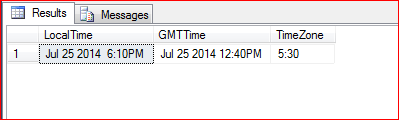# GetDate() V/s GetUTCDate()

Today my colleague asked me, what is the difference between GetDate() and GetUTCDate()? and I thought it would be a nice idea to blog about it. So here it is, my next article.

In simple words the GetDate() returns the local time where as GetUTCDate() returns the GMT time.

GETDATE()

• Returns the current database system timestamp as a datetime value.
• Does not contain the time zone offset.
• The datetime value is derived from the host operating system where the SQL Server is running.

GETUTCDATE()

• It will return the GMT date and time (Greenwich Mean Time).
• GETUTCDATE () can be used to store the timestamp that is independent of time zones.
• The datetime value is derived from the local time and the time zone of the host operating system.

This simple code shows the difference in the GetDate() and GetUTCDate().

```DECLARE @Date DATETIME = GETDATE(),
@UTCDate DATETIME = GETUTCDATE();

WITH CTE
AS (
SELECT @Date AS SYSDATE,
@UTCdate AS UTCDATE,
DATEDIFF(MINUTE, @utcdate, @date) AS DIFF
)
SELECT 'LocalTime' = CONVERT(VARCHAR(20), SYSDATE),
'GMTTime' = CONVERT(VARCHAR(20), UTCDATE),
'TimeZone' = CONVERT(VARCHAR(20), CONVERT(VARCHAR(10), (Diff) / 60)) + ':' +
CONVERT(VARCHAR(2), (ABS(Diff)) % 60)
FROM CTE;
```

Here is the output, the LocaTime shows the server time where as the GMTTime is derived from the local time and the time zone of the host server.Both GetDate() and GetUTCDate() are non-deterministic functions and there are limitation around indexing the columns using there functions. Have a look at BOL as further references.